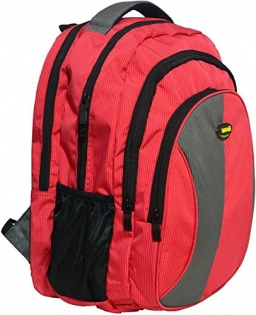# Backpacks 18723

They have two types of backpacks in the store. Blue is for 160 CZK, and red is for 180 CZK. They have 24 backpacks with a total value of CZK 4,120. How many blue and red backpacks do they have?

a =  10
b =  14

### Step-by-step explanation:

a+b=24
160a+180b = 4120

a+b=24
160·a+180·b = 4120

a+b = 24
160a+180b = 4120

Pivot: Row 1 ↔ Row 2
160a+180b = 4120
a+b = 24

Row 2 - 1/160 · Row 1 → Row 2
160a+180b = 4120
-0.13b = -1.75

b = -1.75/-0.125 = 14
a = 4120-180b/160 = 4120-180 · 14/160 = 10

a = 10
b = 14

Our linear equations calculator calculates it.Did you find an error or inaccuracy? Feel free to write us. Thank you!

Tips for related online calculators
Do you have a system of equations and looking for calculator system of linear equations?